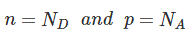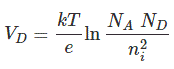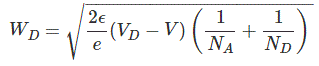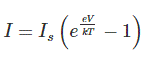# PN Junction Diode and Characteristics of PN Junction Diode

PN junction diode is one of the fundamental elements in electronics. In this type of diode, we dope one side of a semiconductor piece with an acceptor impurity and another side with a donor impurity. A PN junction diode is a two-terminal electronics element, which can be classified as either ‘step graded’ or ‘linear graded’.

In a step graded PN junction diode, the concentration of dopants both, in the N side and the P side are uniform up to the junction. But in a linearly graded junction, the doping concentration varies almost linearly with the distance from the junction. When we do not apply any voltage across the PN diode, free electrons will diffuse through the junction to P side and holes will diffuse through the junction to N side and they combine with each other.

Thus the acceptor atoms in the p-side near the junction edge and donor atoms in n-side near junction edge become negative and positive ions respectively. The existence of negative ions in the p-type side along the junction and positive ions in the n-type side along the junction edge creates an electric field. The electric filed opposes further diffusion of free electrons from the n-type side and holes from the p-type side of the PN junction diode. We call this region across the junction where the uncovered charges (ions) exist, as depletion region.

If, we apply forward bias voltage to the p-n junction diode. That means if the positive side of the battery is connected to the p-side, then the depletion regions width decreases and carriers (holes and free electrons) flow across the junction. If we apply a reverse bias voltage to the diode, the depletion width increases and no charge can flow across the junction.

## P-N Junction Diode Characteristics

Let us consider a pn junction with a donor concentration ND and acceptor concentration NA. Let us also assume that all the donor atoms have donated free electrons and become positive donor ions and all the acceptor atoms have accepted electrons and created corresponding holes and become negative acceptor ions. So we can say the concentration of free electrons (n) and donor ions ND are the same and similarly, the concentration of holes (p) and acceptor ions (NA) are the same. Here, we have ignored the holes and free electrons created in the semiconductors due to unintentional impurities and defects.Across the pn junction, the free electrons donated by donor atoms in n-type side diffuse to the p-typer side and recombine with holes. Similarly, the holes created by acceptor atoms in p-type side diffuse to the n-type side and recombine with free electrons. After this recombination process, there is a lack of or depletion of charge carriers (free electrons and holes) across the junction. The region across the junction where the free charge carriers get depleted is called depletion region. Due to the absence of free charge carriers (free electrons and holes), the donor ions of n-type side and acceptor ions of p-type side across the junction become uncovered. These positive uncovered donor ions towards n-type side adjacent to the junction and negative uncovered acceptors ions towards p-type side adjacent to the junction cause a space charge across the pn junction. The potential developed across the junction due to this space charge is called the diffusion voltage. The diffusion voltage across a pn junction diode can be expressed asThe diffusion potential creates a potential barrier for further migration of free electrons from n-type side to p-type side and holes from p-type side to n-type side. That means diffusion potential prevents charge carriers to cross the junction. This region is highly resistive because of depletion of free charge carriers in this region. The width of the depletion region depends on the applied bias voltage. The relation between the width of the depletion region and bias voltage can be represented by an equation called Poisson Equation.Here, ε is the permittivity of the semiconductor and V is the biasing voltage. So, on an application of a forward bias voltage the width of the depletion region i.e. pn junction barrier decreases and ultimately disappears. Hence, in absence of potential barrier across the junction in the forward bias condition free electrons enter into the p-type region and holes enter into the n-type region, where they recombine and release a photon at each recombination. As a result, there will be a forward current flowing through the diode. The current through the PN junction is expressed asHere, voltage V is applied across the pn junction and total current I, flows through the pn junction. Is is reverse saturation current, e = charge of electron, k is Boltzmann constant and T is temperature in Kelvin scale.

The graph below shows the current-voltage characteristic of a PN junction diode.When, V is positive the junction is forward biased, and when V is negative, the junction is reverse biased. When V is negative and less than VTH, the current is minimal. But when V exceeds VTH, the current suddenly becomes very high. The voltage VTH is known as the threshold or cut in voltage. For Silicon diode VTH = 0.6 V. At a reverse voltage corresponding to the point P, there is abrupt increment in reverse current. This portion of the characteristics is known as breakdown region.

Want To Learn Faster? 🎓
Get electrical articles delivered to your inbox every week.
No credit card required—it’s 100% free.